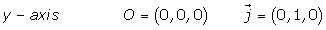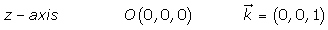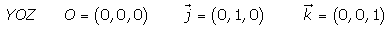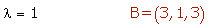Learn from home

The teachers## Exercise 1

Determine the equations of the coordinate axes and the coordinate planes.

## Exercise 2

Determine the equation of the plane that contains the lines:## Exercise 3

Determine the equation of the plane that contains the point A = (2, 5, 1) and the line:## Exercise 4

Find the intersecting point between the plane x + 2y − z − 2 = 0, the line determined by the point (1, −3, 2) and the vector.

## Exercise 5

Determine, in intercept form, the equation of the plane that passes through the points A = (2, 0, 0), B = (0, 4, 0) and C = (0, 0, 7).

## Exercise 6

π is a plane that passes through P = (1, 2, 1) and intersects the positive coordinate semi-axes at points A, B and C. If ABC is an equilateral triangle, determine the equations of π.

## Exercise 7

Find the equation of the plane that passes through the point P = (1, 1, 1) and is parallel to:## Exercise 8

Determine the equation of the plane that contains the lineand is parallel to the line.

## Exercise 9

Calculate the equation of the plane that passes through the point (1, 1, 2) and is parallel to the following lines:## Solution of exercise 1

Determine the equations of the coordinate axes and the coordinate planes.## Solution of exercise 2

Determine the equation of the plane that contains the lines:## Solution of exercise 3

Determine the equation of the plane that contains the point A = (2, 5, 1) and the line:## Solution of exercise 4

Find the intersecting point between the plane x + 2y − z − 2 = 0, the line determined by the point (1, −3, 2) and the vector.## Solution of exercise 5

Determine, in intercept form, the equation of the plane that passes through the points A = (2, 0, 0), B = (0, 4, 0) and C = (0, 0, 7).## Solution of exercise 6

π is a plane that passes through P = (1, 2, 1) and intersects the positive coordinate semi-axes at points A, B and C. If ABC is an equilateral triangle, determine the equations of π.As the triangle is equilateral, the three line segments are equal.## Solution of exercise 7

Find the equation of the plane that passes through the point P = (1, 1, 1) and is parallel to:## Solution of exercise 8

Determine the equation of the plane that contains the lineand is parallel to the line.

The point A = (2, 2, 4) and the vectorbelong to the plane because the line is in the plane.

The vectoris a vector in the plane because it is parallel to the line.## Solution of exercise 9

Calculate the equation of the plane that passes through the point (1, 1, 2) and is parallel to the following lines:Did you like the article?(1 votes, average: 5.00 out of 5)Loading...

Emma

I am passionate about travelling and currently live and work in Paris. I like to spend my time reading, gardening, running, learning languages and exploring new places.

Did you like
this resource?

Bravo!